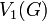# Equal verbal subgroups for a subvariety not implies equal marginal subgroups

## Statement

It is possible to have a group$G$ and two subvarieties$\mathcal{V}_1$ and$\mathcal{V}_2$ of the variety of groups such that the following hold:

•$V_1(G) = V_2(G)$, where$V_1(G)$ and$V_2(G)$ denote respectively the$\mathcal{V}_1$-verbal subgroup and$\mathcal{V}_2$-verbal subgroup of$G$.
•$V_1^*(G) \ne V_2^*(G)$, where$V_1^*(G)$ and$V_2^*(G)$ denote respectively the$\mathcal{V}_1$-marginal subgroup and$\mathcal{V}_2$-marginal subgroup of$G$.

## Proof

### Perfect and centerless

Consider an example where:

•$G$ is a perfect group that is not a centerless group (see perfect not implies centerless), for instance,$G$ is isomorphic to special linear group:SL(2,5).
•$\mathcal{V}_1$ is the variety of abelian groups.
•$\mathcal{V}_2$ is the variety comprising the trivial group.

Then:

•$V_1(G) = [G,G]$ is the derived subgroup of$G$. Since$G$ is perfect,$V_1(G) = G$.
•$V_2(G) = G$.
• Thus,$V_1(G) = V_2(G)$.

However:

•$V_1^*(G)$ is the center of$G$, which is nontrivial since$G$ is not centerless.
•$V_2^*(G)$ is the trivial subgroup of$G$.
• Thus,$V_1^*(G) \ne V_2^*(G)$.

### Example with a finite nilpotent group

Consider the example where:

•$G$ is the central product of D8 and Z4.
•$\mathcal{V}_1$ is the variety of abelian groups.
•$\mathcal{V}_2$ is the variety of groups of exponent two (along with the trivial group).

Then:

•$V_1(G)$ is the derived subgroup of$G$, and is isomorphic to cyclic group:Z2.
•$V_2(G)$ is the Frattini subgroup of$G$, and it coincides with the derived subgroup of$G$.
•$V_1^*(G)$ is the center of$G$, and it is isomorphic to cyclic group:Z4.
•$V_2^*(G) = \Omega_1(Z(G))$ is the socle of$G$ (see socle equals Omega-1 of center in nilpotent p-group), and it in fact coincides with$V_1(G) = V_2(G)$. In particular, it is not the same as$V_1^*(G)$.